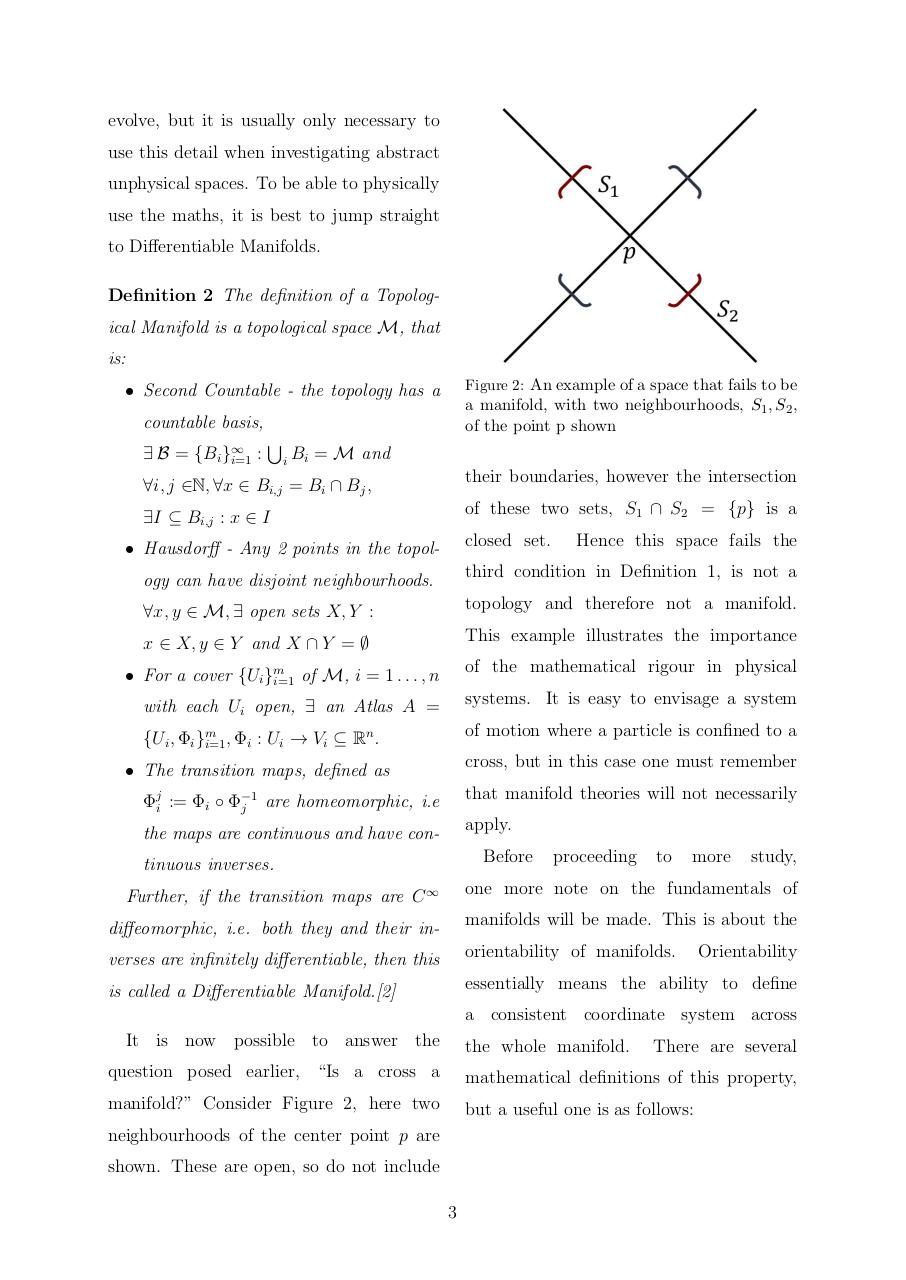# Noether's Theorem on Non Trivial Manifolds By Daniel Martin.pdfPage 1 2 34520

#### Text preview

evolve, but it is usually only necessary to
use this detail when investigating abstract
unphysical spaces. To be able to physically
use the maths, it is best to jump straight
to Differentiable Manifolds.
Definition 2 The definition of a Topological Manifold is a topological space M, that
is:
Figure 2: An example of a space that fails to be

• Second Countable - the topology has a

a manifold, with two neighbourhoods, S1 , S2 ,
of the point p shown

countable basis,
∃ B = {Bi }∞
i=1 :

S

i

Bi = M and

∀i, j ∈N, ∀x ∈ Bi,j = Bi ∩ Bj ,

their boundaries, however the intersection

∃I ⊆ Bi,j : x ∈ I

of these two sets, S1 ∩ S2 = {p} is a
closed set.

• Hausdorff - Any 2 points in the topol-

Hence this space fails the

ogy can have disjoint neighbourhoods.

third condition in Definition 1, is not a

∀x, y ∈ M, ∃ open sets X, Y :

topology and therefore not a manifold.

x ∈ X, y ∈ Y and X ∩ Y = ∅

This example illustrates the importance

• For a cover {Ui }m
i=1 of M, i = 1 . . . , n

of the mathematical rigour in physical

with each Ui open, ∃ an Atlas A =

systems. It is easy to envisage a system
of motion where a particle is confined to a

n
{Ui , Φi }m
i=1 , Φi : Ui → Vi ⊆ R .

cross, but in this case one must remember

• The transition maps, defined as
Φji := Φi ◦ Φ−1
are homeomorphic, i.e
j

that manifold theories will not necessarily

the maps are continuous and have con-

apply.
Before

tinuous inverses.

proceeding

to

more

study,

Further, if the transition maps are C ∞

one more note on the fundamentals of

diffeomorphic, i.e. both they and their in-

verses are infinitely differentiable, then this

orientability of manifolds.

is called a Differentiable Manifold.

essentially means the ability to define

Orientability

a consistent coordinate system across
It is now possible to answer the

the whole manifold.

There are several

question posed earlier, “Is a cross a

mathematical definitions of this property,

manifold?” Consider Figure 2, here two

but a useful one is as follows:

neighbourhoods of the center point p are
shown. These are open, so do not include
3